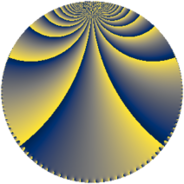# Properties

 Label 384.4.fLevel $384$ Weight $4$ Character orbit 384.f Rep. character $\chi_{384}(191,\cdot)$ Character field $\Q$ Dimension $48$ Newform subspaces $9$ Sturm bound $256$ Trace bound $15$

# Related objects

## Defining parameters

 Level: $$N$$ $$=$$ $$384 = 2^{7} \cdot 3$$ Weight: $$k$$ $$=$$ $$4$$ Character orbit: $$[\chi]$$ $$=$$ 384.f (of order $$2$$ and degree $$1$$) Character conductor: $$\operatorname{cond}(\chi)$$ $$=$$ $$24$$ Character field: $$\Q$$ Newform subspaces: $$9$$ Sturm bound: $$256$$ Trace bound: $$15$$ Distinguishing $$T_p$$: $$5$$, $$23$$

## Dimensions

The following table gives the dimensions of various subspaces of $$M_{4}(384, [\chi])$$.

Total New Old
Modular forms 208 48 160
Cusp forms 176 48 128
Eisenstein series 32 0 32

## Trace form

 $$48 q + O(q^{10})$$ $$48 q + 1200 q^{25} - 464 q^{33} - 912 q^{49} + 688 q^{57} - 864 q^{73} + 624 q^{81} + 6144 q^{97} + O(q^{100})$$

## Decomposition of $$S_{4}^{\mathrm{new}}(384, [\chi])$$ into newform subspaces

Label Dim $A$ Field CM Traces $q$-expansion
$a_{2}$ $a_{3}$ $a_{5}$ $a_{7}$
384.4.f.a $4$ $22.657$ $$\Q(\sqrt{2}, \sqrt{-3})$$ $$\Q(\sqrt{-6})$$ $$0$$ $$0$$ $$0$$ $$0$$ $$q+\beta _{2}q^{3}-7\beta _{1}q^{5}-\beta _{3}q^{7}-3^{3}q^{9}+\cdots$$
384.4.f.b $4$ $22.657$ $$\Q(\zeta_{8})$$ $$\Q(\sqrt{-2})$$ $$0$$ $$0$$ $$0$$ $$0$$ $$q-\zeta_{8}^{2}q^{3}+(-23+5\zeta_{8})q^{9}+(3\zeta_{8}^{2}+\cdots)q^{11}+\cdots$$
384.4.f.c $4$ $22.657$ $$\Q(\zeta_{8})$$ None $$0$$ $$0$$ $$0$$ $$0$$ $$q+(-\zeta_{8}+2\zeta_{8}^{2})q^{3}-2\zeta_{8}^{2}q^{5}+2\zeta_{8}^{3}q^{7}+\cdots$$
384.4.f.d $4$ $22.657$ $$\Q(\zeta_{8})$$ None $$0$$ $$0$$ $$0$$ $$0$$ $$q+(\zeta_{8}-2\zeta_{8}^{2})q^{3}-2\zeta_{8}^{2}q^{5}-2\zeta_{8}^{3}q^{7}+\cdots$$
384.4.f.e $4$ $22.657$ $$\Q(i, \sqrt{26})$$ None $$0$$ $$0$$ $$0$$ $$0$$ $$q+(-\beta _{1}-\beta _{2})q^{3}+(\beta _{1}+2\beta _{2})q^{5}+\beta _{3}q^{7}+\cdots$$
384.4.f.f $4$ $22.657$ $$\Q(i, \sqrt{26})$$ None $$0$$ $$0$$ $$0$$ $$0$$ $$q+\beta _{2}q^{3}+(\beta _{1}+2\beta _{2})q^{5}+\beta _{3}q^{7}+(5^{2}+\cdots)q^{9}+\cdots$$
384.4.f.g $8$ $22.657$ 8.0.$$\cdots$$.1 None $$0$$ $$0$$ $$0$$ $$0$$ $$q+(\beta _{3}+\beta _{4})q^{3}+(-2\beta _{3}+\beta _{6})q^{5}+(-3\beta _{2}+\cdots)q^{7}+\cdots$$
384.4.f.h $8$ $22.657$ 8.0.$$\cdots$$.1 None $$0$$ $$0$$ $$0$$ $$0$$ $$q+\beta _{4}q^{3}+(-2\beta _{3}+\beta _{6})q^{5}+(-3\beta _{2}+\cdots)q^{7}+\cdots$$
384.4.f.i $8$ $22.657$ 8.0.$$\cdots$$.6 None $$0$$ $$0$$ $$0$$ $$0$$ $$q+(-\beta _{1}-\beta _{2})q^{3}+\beta _{3}q^{5}+\beta _{7}q^{7}+\cdots$$

## Decomposition of $$S_{4}^{\mathrm{old}}(384, [\chi])$$ into lower level spaces

$$S_{4}^{\mathrm{old}}(384, [\chi]) \cong$$ $$S_{4}^{\mathrm{new}}(24, [\chi])$$$$^{\oplus 5}$$$$\oplus$$$$S_{4}^{\mathrm{new}}(96, [\chi])$$$$^{\oplus 3}$$$$\oplus$$$$S_{4}^{\mathrm{new}}(192, [\chi])$$$$^{\oplus 2}$$